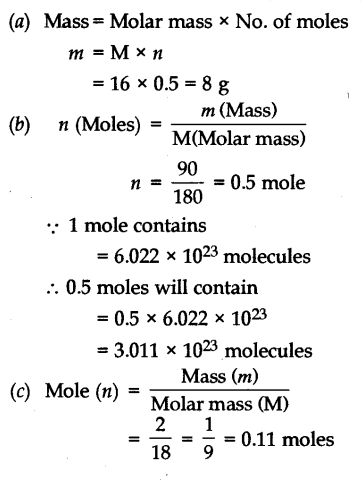# (a) Calculate the mass of 0.5 mole of oxygen atoms. (b) Calculate the number of molecules of glucose present in its 90 grams (molecular mass of glucose is 180 u) (c) Calculate number of moles of water in 2 grams of water. Atomic mass of H = 1 u, O = 16 u